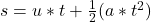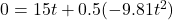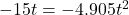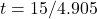Question

A soccer ball kicked on a level field has an initial vertical velocity component of 15.0 mps. assuming the ball lands at the same height from which it was kicked was the total time of the ball in the air?

1.The time taken for the ball to fly up in the air and back down again is 3.058 seconds.

Explanation:

Since the ball ends up at the same vertical distance ( on the ground) as it was at the start of its motion, we can set the total displacement of the ball equal to 0.

Thus, this problem can be simply solved by the following equation of motion:Here, s = total change in distance = 0 m

u = initial speed = 15 m/s

a = acceleration due to gravity = 9.81 m/s^2

t = time (to be found)

Substituting these values in the equation we get:t = 3.058 seconds

So, the time taken for the ball to fly up in the air and back down again is 3.058 seconds.